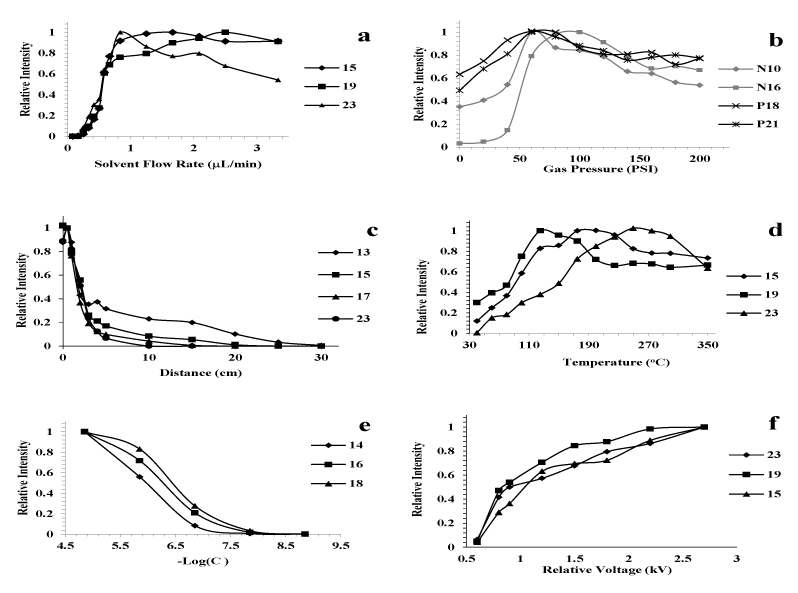Figure 6: Dependence of signal intensity of myoglobin ions on (a) solvent flow rate, (b) gas pressure (PSI), (c) spray tip to MS inlet distance, (d) temperature (°C), (e) concentration (mol/L) and (f) relative voltage (kV). In (b), N10 and N16 represent negative ions with 10 and 16 charges respectively, while P18 and P21 represent positive ions with 18 and 21 charges respectively. In the remaining figures all the selected charge states are for positive ions.
Goto home»